Chapter 10 Rotational Motion and Angular Momentum

# 10.7 Gyroscopic Effects: Vector Aspects of Angular Momentum

### Summary

• Describe the right-hand rule to find the direction of angular velocity, momentum, and torque.
• Explain the gyroscopic effect.
• Study how Earth acts like a gigantic gyroscope.

Angular momentum is a vector and, therefore, has direction as well as magnitude. Torque affects both the direction and the magnitude of angular momentum. What is the direction of the angular momentum of a rotating object like the disk in Figure 1? The figure shows the right-hand rule used to find the direction of both angular momentum and angular velocity. Both$\textbf{L}$and$\boldsymbol{\omega}$are vectors—each has direction and magnitude. Both can be represented by arrows. The right-hand rule defines both to be perpendicular to the plane of rotation in the direction shown. Because angular momentum is related to angular velocity by$\boldsymbol{\textbf{L}=I\omega},$the direction of$\textbf{L}$is the same as the direction of$\boldsymbol{\omega}.$Notice in the figure that both point along the axis of rotation.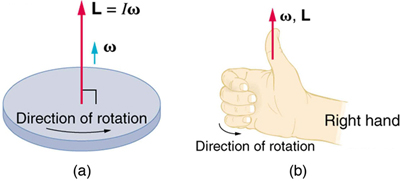Figure 1. Figure (a) shows a disk is rotating counterclockwise when viewed from above. Figure (b) shows the right-hand rule. The direction of angular velocity ω size and angular momentum L are defined to be the direction in which the thumb of your right hand points when you curl your fingers in the direction of the disk’s rotation as shown.

Now, recall that torque changes angular momentum as expressed by

$\boldsymbol{\textbf{net }\tau\:=}$$\boldsymbol{\frac{\Delta\textbf{L}}{\Delta{t}}}.$

This equation means that the direction of$\boldsymbol{\Delta\textbf{L}}$is the same as the direction of the torque$\boldsymbol{\tau}$that creates it. This result is illustrated in Figure 2, which shows the direction of torque and the angular momentum it creates.

Let us now consider a bicycle wheel with a couple of handles attached to it, as shown in Figure 3. (This device is popular in demonstrations among physicists, because it does unexpected things.) With the wheel rotating as shown, its angular momentum is to the woman’s left. Suppose the person holding the wheel tries to rotate it as in the figure. Her natural expectation is that the wheel will rotate in the direction she pushes it—but what happens is quite different. The forces exerted create a torque that is horizontal toward the person, as shown in Figure 3(a). This torque creates a change in angular momentum$\textbf{L}$in the same direction, perpendicular to the original angular momentum$\textbf{L},$thus changing the direction of$\textbf{L}$but not the magnitude of$\textbf{L}.$Figure 3 shows how$\boldsymbol{\Delta\textbf{L}}$and$\textbf{L}$add, giving a new angular momentum with direction that is inclined more toward the person than before. The axis of the wheel has thus moved perpendicular to the forces exerted on it, instead of in the expected direction.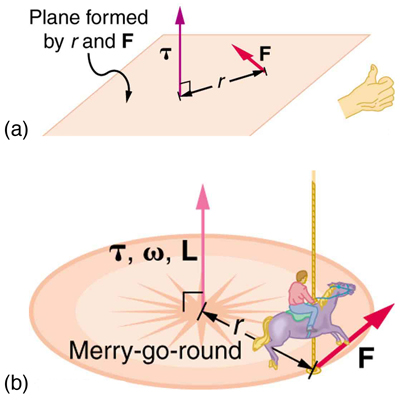Figure 2. In figure (a), the torque is perpendicular to the plane formed by r and F and is the direction your right thumb would point to if you curled your fingers in the direction of F. Figure (b) shows that the direction of the torque is the same as that of the angular momentum it produces.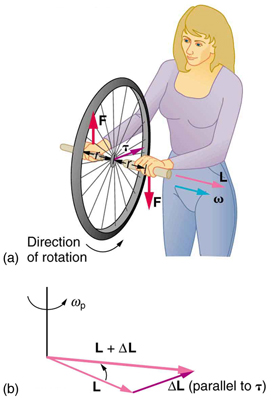Figure 3. In figure (a), a person holding the spinning bike wheel lifts it with her right hand and pushes down with her left hand in an attempt to rotate the wheel. This action creates a torque directly toward her. This torque causes a change in angular momentum ΔL in exactly the same direction. Figure (b) shows a vector diagram depicting how ΔL and L add, producing a new angular momentum pointing more toward the person. The wheel moves toward the person, perpendicular to the forces she exerts on it.

This same logic explains the behavior of gyroscopes. Figure 4 shows the two forces acting on a spinning gyroscope. The torque produced is perpendicular to the angular momentum, thus the direction of the torque is changed, but not its magnitude. The gyroscope precesses around a vertical axis, since the torque is always horizontal and perpendicular to$\textbf{L}.$If the gyroscope is not spinning, it acquires angular momentum in the direction of the torque ($\boldsymbol{\textbf{L}=\Delta\textbf{L}}$), and it rotates around a horizontal axis, falling over just as we would expect.

Earth itself acts like a gigantic gyroscope. Its angular momentum is along its axis and points at Polaris, the North Star. But Earth is slowly precessing (once in about 26,000 years) due to the torque of the Sun and the Moon on its nonspherical shape.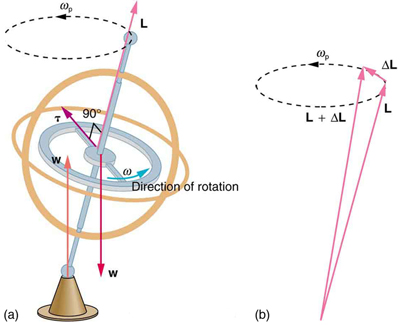Figure 4. As seen in figure (a), the forces on a spinning gyroscope are its weight and the supporting force from the stand. These forces create a horizontal torque on the gyroscope, which create a change in angular momentum ΔL that is also horizontal. In figure (b), ΔL and L add to produce a new angular momentum with the same magnitude, but different direction, so that the gyroscope precesses in the direction shown instead of falling over.

1: Rotational kinetic energy is associated with angular momentum? Does that mean that rotational kinetic energy is a vector?

# Section Summary

• Torque is perpendicular to the plane formed by$\boldsymbol{r}$and$\textbf{F}$and is the direction your right thumb would point if you curled the fingers of your right hand in the direction of$\textbf{F}.$The direction of the torque is thus the same as that of the angular momentum it produces.
• The gyroscope precesses around a vertical axis, since the torque is always horizontal and perpendicular to$\textbf{L}.$If the gyroscope is not spinning, it acquires angular momentum in the direction of the torque ($\boldsymbol{\textbf{L}=\Delta\textbf{L}}$), and it rotates about a horizontal axis, falling over just as we would expect.
• Earth itself acts like a gigantic gyroscope. Its angular momentum is along its axis and points at Polaris, the North Star.

### Conceptual Questions

1: While driving his motorcycle at highway speed, a physics student notices that pulling back lightly on the right handlebar tips the cycle to the left and produces a left turn. Explain why this happens.

2: Gyroscopes used in guidance systems to indicate directions in space must have an angular momentum that does not change in direction. Yet they are often subjected to large forces and accelerations. How can the direction of their angular momentum be constant when they are accelerated?

### Problems & Exercises

1: Integrated Concepts

The axis of Earth makes a 23.5° angle with a direction perpendicular to the plane of Earth’s orbit. As shown in Figure 5, this axis precesses, making one complete rotation in 25,780 y.

(a) Calculate the change in angular momentum in half this time.

(b) What is the average torque producing this change in angular momentum?

(c) If this torque were created by a single force (it is not) acting at the most effective point on the equator, what would its magnitude be?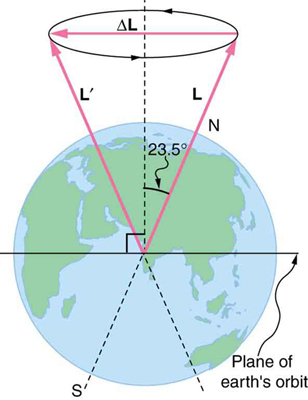Figure 5. The Earth’s axis slowly precesses, always making an angle of 23.5° with the direction perpendicular to the plane of Earth’s orbit. The change in angular momentum for the two shown positions is quite large, although the magnitude L is unchanged.

## Glossary

right-hand rule
direction of angular velocity ω and angular momentum L in which the thumb of your right hand points when you curl your fingers in the direction of the disk’s rotation

### Solutions

1: No, energy is always a scalar whether motion is involved or not. No form of energy has a direction in space and you can see that rotational kinetic energy does not depend on the direction of motion just as linear kinetic energy is independent of the direction of motion.

Problems & Exercises

1:

(a)$\boldsymbol{5.64\times10^{33}\textbf{ kg}\cdotp\textbf{ m}^2\textbf{/s}}$

(b)$\boldsymbol{1.39\times10^{22}\textbf{ N}\cdotp\textbf{ m}}$

(c)$\boldsymbol{2.17\times10^{15}\textbf{ N}}$